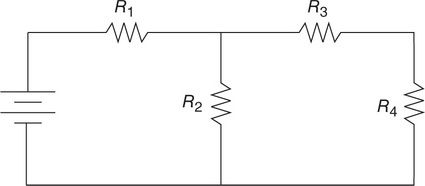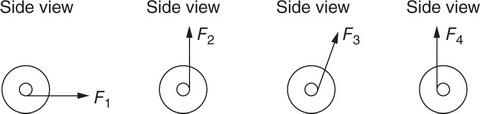# AP Physics 1 Multiple-select Practice Test 2

### Test Information9 questions18 minutes

1. A sound wave travels through a metal rod with wavelength λ and frequency f. Which of the following is true? Select two answers.

2. The distance between the centers of two objects is d. Each object has identical mass m and identical charge –q. Choose all of the correct statements about the similarities and differences between the electric and gravitational force between the two objects. Select two answers.

3.Which placement of voltmeters will allow for determination of the voltage across resistor R2 in the circuit diagrammed in the preceding figure? Select two answers.

4. A student of mass 50 kg stands on a scale in an elevator. The scale reads 800 N. Which of the following could describe how the elevator is moving? Select two answers.

5. A 1-m-long pipe is closed at one end. The speed of sound in the pipe is 300 m/s. Which of the following frequencies will resonate in the pipe? Select two answers.

6.The device shown in the preceding figure consists of two wheels connected by a thick axle. A force can be applied to the axle by pulling a rope at several positions along the axle. Assuming the spool does not slip on the table, which of the pictured applied forces would cause rotation to the right of the device’s wheels? Select two answers.7. Which of the experiments listed here measure inertial mass? Select two answers.

8. An object on a spring vibrates in simple harmonic motion. A sonic motion detector is placed under the object. Which of the following determines the period of the object’s oscillation? Select two answers.

9. Two carts on a negligible-friction surface collide with each other. Which of the following is a correct statement about an elastic collision between the carts? Select two answers.